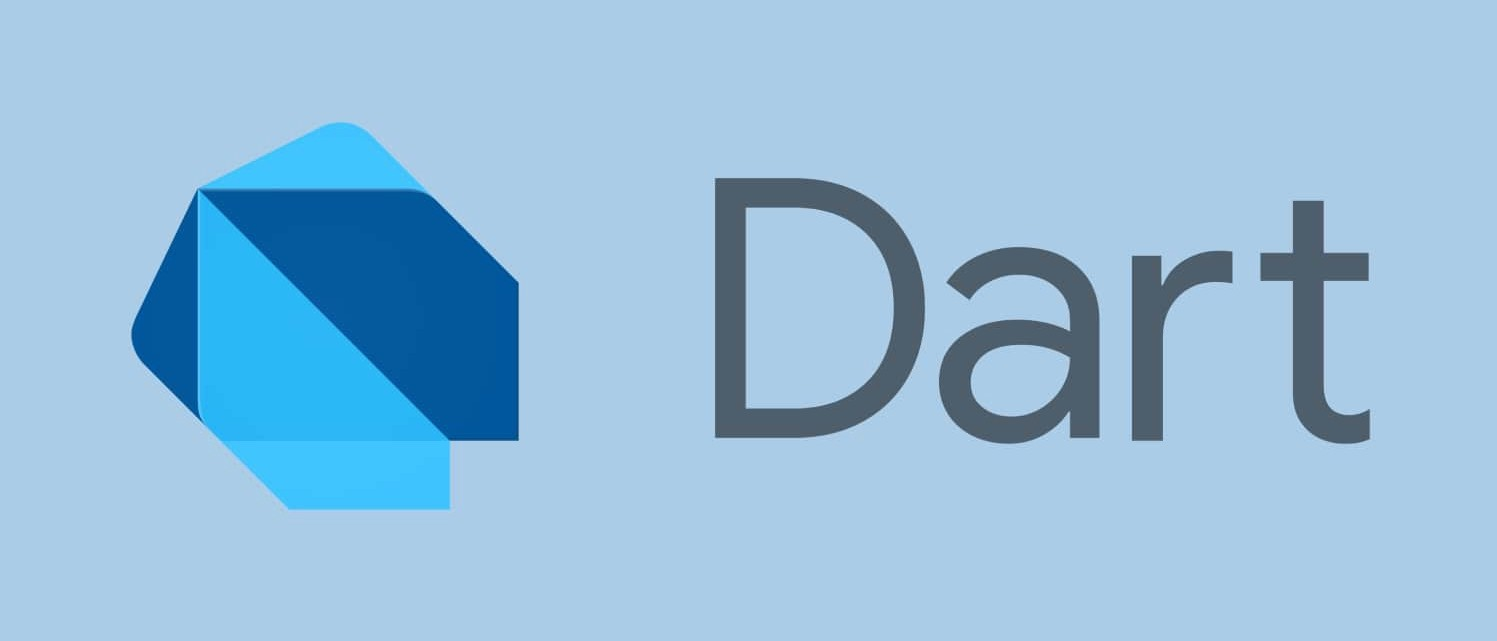# Boolean in Dart #

Let’s understand this using life example. In school life we have seen True/False kind of question, where we have to tell weather the statement is true or false.

Boolean came from there only. Boolean is used of that kind of purpose only. Whenever you need answer in True/False then we use boolean.

Boolean data can have at most two possible values.

1. true
2. false

In dart `boolean` is refer as `bool`

### How to declare and assign value of bool #main.dart
``````void main(){
bool aa = true; // Assign value while declaring the variable

bool yy ;       // declare a variable
yy = false;     // let's assign some value to variable

print(ans);
print(yy);

aa = false;     // update value of bool type
print(aa);
}
``````

### Real Example #main.dart
``````void main(){
int a = 10;
int b = 20;
boole answer = a < b;   // Yes, A is less than B
print(answer);          // We will get true in console
}
``````